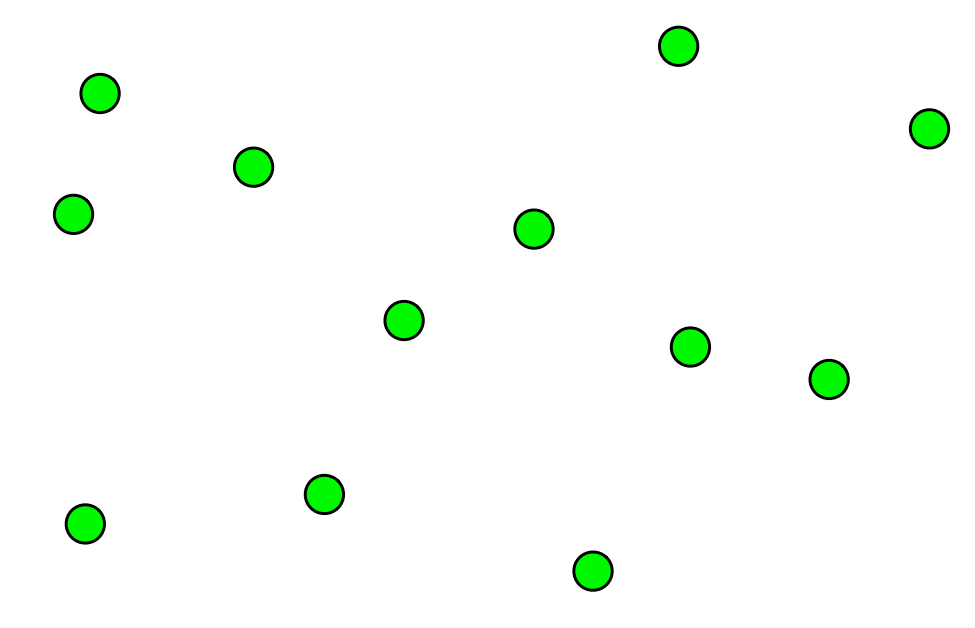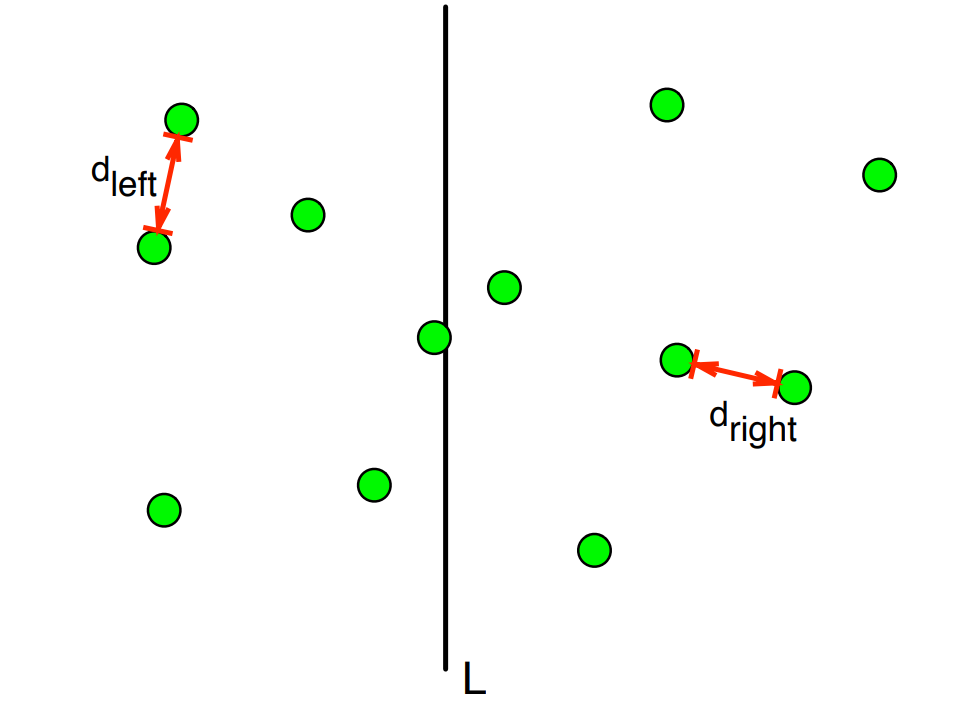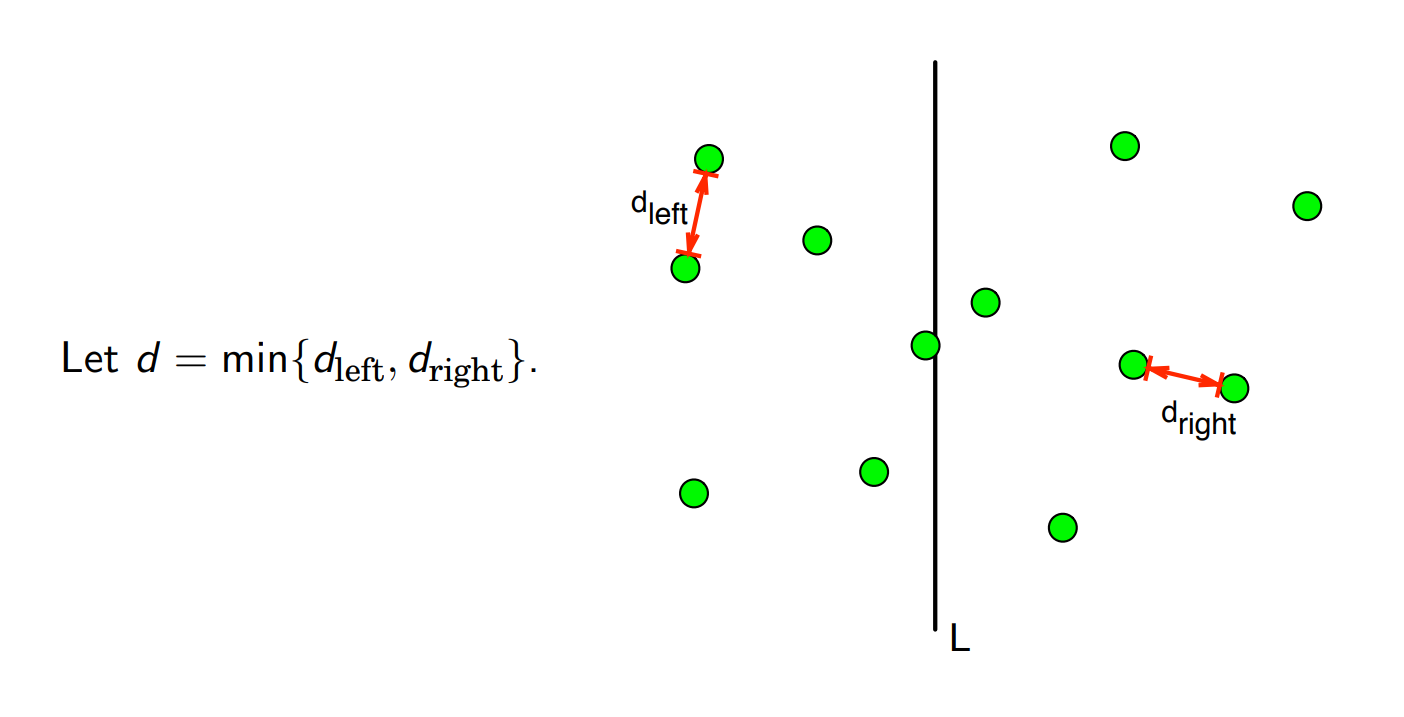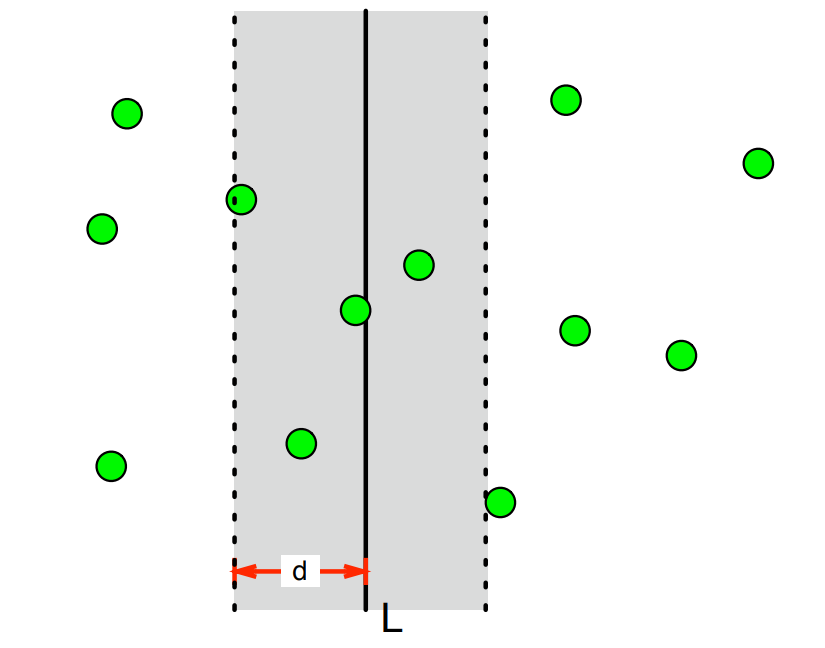×

Search anything:

# Closest Pair of Points

#### Algorithms computational geometry divide and conquerGet this book -> Problems on Array: For Interviews and Competitive Programming

In this article, we have explored different algorithms using which we can find the Closest Pair of Points in a given set of N points efficiently in O(N logN) time. This involves the idea of Divide and Conquer.

• Introduction
• Brute Force
• Divide and Conquer
• Optimised Divide and Conquer
• Overview

# Introduction

Finding the closest point to another in a given graph is a simple sounding problem which can get quite complex in trying to solve efficiently, in this article we will discuss 3 ways of solving this problem, with running times of O(n^2), O(nlogn^2) and O(nlogn).

# Method 1: Brute Force

We can naively solve this problem by simply computing the distance between each point and the starting point, and then comparing them to find the shortest distance which is then returned. The problem with this approach is that it becomes inefficient with a large amount of data leaving it in polynominal time since we are computing each distance which takes O(n) time and there are n items we have to do this for, leaving us in O(n^2).

``````import math

class Point():
def __init__(self, x, y):
self.x = x
self.y = y

def find_distance(p1, p2):
return math.sqrt((p1.x - p2.x)**2 + (p1.y - p2.y)**2)

def brute_force(points, n):
minimum = float('inf')
for i in range(n):
for j in range(i + 1, n):
if find_distance(points[i], points[j]) < minimum:
minimum = find_distance(points[i], points[j])

return minimum

points = [Point(5,2), Point(2,7), Point(10,16),
Point(34,50), Point(3,6), Point(20,5),
Point(12,4), Point(8,9), Point(5,21)]

n = len(points)

print(brute_force(points, n))
``````

# Method 2: Divide and Conquer

We can reduce the time complexity of our approach by implementing a divide and conquer algorithm in order to minimise the amount of points we are searching for at one time. This is in O(nlogn^2) time, which we will optimisise further in the next method 3. The process for this approach is as follows:

``````Input: Array of points arr[]
* Find middle point of sorted array
* Divide array into 2 halves, one is all elements before and up to the midpoint and the second is all elements after the midpoint
* Find the smallest distance in both the sub-arrays reccursively, left (i) and right (j)
* Find the minimum of the left and right (k)
* We have the upper and lower bound of the minimum point now (i and j)
* We now know that one point is in the left half and the other is in the right so we can find all the points with x coordinates closer to k than i and j, we then build an array with these points l
* Sort this array in relation to their y coordinates
* Find the smallest distance in l
* Return the minimum of k and smallest distance from l
``````
``````import math
import copy

class Point():
def __init__(self, x, y):
self.x = x
self.y = y

def find_dist(p1, p2):
return math.sqrt((p1.x - p2.x)**2 + (p1.y - p2.y)**2)

def brute_force(points, n):
minimum = float('inf')
for i in range(n):
for j in range(i + 1, n):
if find_dist(points[i], points[j]) < minimum:
minimum = find_dist(points[i], points[j])

return minimum

def closest_list(list, size, k):

minimum = k

for i in range(size):
j = i + 1
while j < size and (list[j].y -
list[i].y) < minimum:
minimum = find_dist(list[i], list[j])
j += 1

return minimum

def find_closest(points, points_close, n):

if n <= 3:
return brute_force(points, n)

mid = n // 2
midpoint = points[mid]

pointsleft = points[:mid]
pointsright = points[mid:]

kleft = find_closest(pointsleft, points_close, mid)
kright = find_closest(pointsright, points_close, n - mid)

k = min(kleft, kright)

list_points = []
list_close = []
lr = pointsleft + pointsright
for i in range(n):
if abs(lr[i].x - midpoint.x) < k:
list_points.append(lr[i])
if abs(points_close[i].x - midpoint.x) < k:
list_close.append(points_close[i])

list_points.sort(key = lambda point: point.y)
min1 = min(k, closest_list(list_points, len(list_points), k))
min2 = min(k, closest_list(list_close, len(list_close), k))

return min(min1,min2)

def closest(points, n):
points.sort(key = lambda point: point.x)
points_close = copy.deepcopy(points)
points_close.sort(key = lambda point: point.y)

return find_closest(points, points_close, n)

points = [Point(20, 4), Point(9, 8), Point(5, 10), Point(4, 4), Point(2, 0), Point(18, 21)]
n = len(points)

print(closest(points, n))
``````

We can compute the time complexity for our algorithm as such:

• We divide the points into 2 sub-arrays and then find the closest points based on the bounds calculated, placed into an array: O(n)
• Sorts new array: O(nlogn)
• Find closest points: O(n)

Total = O(nlogn^2)

# Method 3: D&C Optimised

We can optimise our above method in sorting step. Currently sorting l takes O(nlogn) time however if we recursively sort and merge, we reduce this to linear time. The processes of the algorithm is otherwise the same apart from this stage. Reducing our final complexity to O(nlogn).

``````import math

points = [(20, 4), (9, 8), (5, 10), (4, 4), (2, 0), (18, 21)]

def merge_sort(arr, coord):

if len(arr) == 1:
return arr

mid = len(arr) // 2
pointsleft = arr[:mid]
pointsright = arr[mid:]

left_sorted = merge_sort(pointsleft, coord)
right_sorted = merge_sort(pointsright, coord)
cmbo = merge(left_sorted,right_sorted, coord)
return cmbo

def merge(A, B, coord):

i = j = 0
C = []

if coord == 'x':
coord = 0
elif coord == 'y':
coord = 1

while i < len(A) and j < len(B):
if A[i][coord] <= B[j][coord]:
C.append(A[i])
i += 1

elif B[j][coord] < A[i][coord]:
C.append(B[j])
j += 1

while i < len(A) and j == len(B):
C.append(A[i])
i += 1

while j < len(B) and i == len(A):
C.append(B[j])
j += 1

return C

def first_sort(points):
Px = merge_sort(points, 'x')
Py = merge_sort(points, 'y')
return Px,Py

Px, Py = first_sort(points)

def find_dist(p1, p2):
return math.sqrt((p1 - p2)**2 + (p1 - p2)**2)

def brute_force(arr):
size = len(arr)
minimum_distance = find_dist(arr, arr)
target_pair = (arr, arr)

if len(arr) == 2:
return find_dist(arr, arr), arr, arr

for i in range(0,size):
for j in range(i+1,size):
distance = find_dist(arr[i], arr[j])
if distance < minimum_distance:
minimum_distance = distance
target_pair = (arr[i], arr[j])

return minimum_distance, target_pair

def closest_pair(Px,Py):

if len(Px) <= 3:
return brute_force(Px)

midpoint_x = len(Px) // 2
Qx = Px[:midpoint_x]
Rx = Px[midpoint_x:]
median_x = Px[midpoint_x]
Qy,Ry = [], []

for point in Py:
if point < int(median_x):
Qy.append(point)
else:
Ry.append(point)

min_distance_left = closest_pair(Qx, Qy)
min_distance_right = closest_pair(Rx, Ry)
min_distance = min(min_distance_left, min_distance_right)
x_bar = Qx[-1]
Sy = []

for y in Py:
if x_bar - min_distance < y < x_bar + min_distance:
Sy.append(y)

for i in range(len(Sy) - 1):
for j in range(i+1, min(i + 7, len(Sy))):
points = Sy[i]
points_close = Sy[j]
dist = find_dist(points, points_close)

if dist < min_distance:
min_distance = (dist, points, points_close)

return min_distance

print(closest_pair(Px,Py))
``````

# Explanation

To explain our approach, we can say that we have a set of points (P) and we want to find pair Pi and Pj which are closest togethger:We then divide space in half, and find the closest pair on the left and right side recursively.Since we know that these points are the closest on each side, we can find the minimum by merging together, and then finding the minimum distance between each.Therefore "d" might be the closest pair, however we then need to consider a pair that spreads across our line.So we know if there is a pair with a distance smaller than "d" which is split by the line, then both points must be within "d" distance of the line.

We can then create an array of the points within that region, and sort it by y-coordinates. Therefore we can deduce that if any points in our array are close enough in the space, they are close enough in the array.

We can then finally return the minimum ditance between the two closest points in our created array, and the initial minimum distance we found outside the region, giving us our shortest distance in the whole plane.

To prove the time complexity for this approach:

• We divide the points into 2 sub-arrays and then find the closest points based on the bounds calculated, placed into an array: O(n)
• Split new array according to middle line: O(n)
• Sorting: O(nlogn)
• Find closest points: O(n)

Total = O(nlogn)

# Overview

In this article at OpenGenus, we have explained various ways in solving the "Closest Pair of Points" problem, mainly using divide and conquer methods. We have also gone over the time complexity for each approach and how best to go about optimising the solution.